# Unknown number 17

Milada said, I am thinking of a number such that I evaluate expression x1/3, the value of the expression would be 5. Which number Milada thinking?

x =  125

### Step-by-step explanation: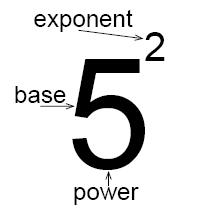Did you find an error or inaccuracy? Feel free to write us. Thank you!## Related math problems and questions:

• Unknown number 4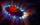I think number. When I divided its square root by its 1/9, I get a number 1. What number am I thinking?
• Unknown number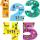Determine the unknown number, which double of its fourth square is equal the fifth its square.
• Evaluate 17Evaluate 2x+6y when x=- 4/5 and y=1/3. Write your answer as a fraction or mixed number in simplest form.
• Unknown number 7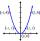Calculate unknown number whose 12th power when divided by the 9th power get a number 27 times greater than the unknown number. Determine the unknown number.
• Unknown number 5I think of an unknown number. If we enlarge it five times, then subtract 3, and the result decreases by 75%, we get one greater than the number. What number am I thinking of?
• Evaluate expressionEvaluate expression using BODMAS rule: 1 1/4+1 1/5÷3/5-5/8
• Value of expressionX=2, y=-5 and z=3 what is the value of x-2y?
• Evaluate valueEvaluate value of the following expressions: 1. The product of nine and a number 12, decreased by six 2. Eight decreased by three times a number 2. 3. The quotient of twice a number 49 and seven.
• Unknown numberFind an unknown number of which 1/5 is 40 greater than one-tenth of that number.
• Meanif the mean of the set of data 5, 17, 19, 14, 15, 17, 7, 11, 16, 19, 5, 5, 10, 8, 13, 14, 4, 2, 17, 11, x is -91.74, what is the value of x?
• Contract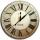8 employees complete the contract for 65 hours. After 17 hours, 3 employees had to leave for another job. How many more hours will the job on contract be fulfilled?
• Two thirdsTwo thirds of the unknown number is 8/7. What is 7/3 of this number?
• TranslateTranslate the following mathematical statement into an algebraic expression or equation. 1. Nine less that the quotient of a number and 3 2. The ratio of 3 and the sum of 4 and an unknown number 3. Eighty is the product of 4 and g 4. The sum of x and 18 i
• Missing digitIf 4x/6x + 5/17 = 1, what digit does the x represents
• Square Number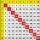If to a square of integer number add 17, we get the square of the next integer number. What is the original number?
• Multiplicative inverse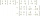Explain why the reciprocal of - 1 1/17 is the multiplicative inverse of - 17/18.
• SelectSelect the statement that describes this expression: 5 x (3 + 4) - 4. a) 5 times the sum of 3 and 4, then subtract 4 b) 5 times 3 plus 4 minus 4 c) 5 more than the sum of 3 and 4, and then subtract 4 d) 5 times 3 plus the difference of 3 and 4 What is the